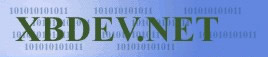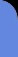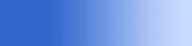Sunday July 12, 2020
 home | about | contact | DonationsSlice of Java. Its simple and its powerful...

Camera - so we can move around

by Ben Kenwright

Yes its cool to create amazing 3D demo's, rotating cubes, 3d teapots - but you want to be able to create more objects and place them in your virtual world...then move around and go closer and rotate around them!  What am I talking about?  Well a camera setup - so in essence we can create a 3D world and move around it.

Believe me, its not that hard to do a basic camera setup...a lot of the times its complicated with matrix's and lots and lots of code for calcuating all sorts of things, like clipping and frustrum which make the camera code look even more complex.

For example, lets say we have a simple cube or sphere for example - no if we add a value to all the z points...e.g...zinc - then our object will seem to move away...and if we subtract zinc from all the points, the z value will move closer to us.....this is a simple forward backward motion.  Sometimes refereed to as 'translation'.  The difference with camera translation though, is everything is in reverse.  As if we want our camera...which is move things closer to the screen, so they get bigger, we subtract a z value from all our vertices.  Assuming a left handed system, where positive z is into the screen.  So just dealing with x, y and z...and not mixing in the rotation things yet...we can straff forward, backward or up and down, by simply adding or subtracting a value from our vertices values.

In most games and books, it tells us not to harm or modify our original data - so you usually have two sets - a master copy and a translated version.  You could keep as many different copies of the data for the different stages of the 3D pipeline that where building, but it takes up lots of space...and we want to keep it simple :).

 Simple Camera Movement (with No Rotation) float x, y, z;       // These would be our original values    float tx,ty,tz;      // Our translated - new values    float movx,movy,movz // These are our precious camera movement info, telling us                         // the current position of our camera.       // First copy our values over    tx = x;    ty = y;    tz = z;      // Now our movement!  Simple forward, back or left right (with no rotation involved)    tx -= movx;    ty -= movy;    tz -= movz;

Hey, now thats simple...but we can only see forward!  We are moving around our 3D world, but we're always looking into the positive z axis.  Now we can do better than that can't we :)  So now comes our rotation equations...which at first are a bit scary, especially if your trig and maths are a bit rusty...but hey, you usually lock these baby's away in a function and use them when you need them.  And I found, that after a while, they sort of start to make sense in a way..hehe....well I did the trig and prooved them...but sometimes it takes time for you to truly get a feel for 3D trig....but if you stick with it, it comes to you in time :)

 Reminder of the Euler Rotation Equations: // ax, ay, and az our the angles of rotation :)    // Rotation about the x-axis    rx = x;    ry = y*cos(ax) - z*sin(ax)    rz = y*sin(ax) + z*cos(ax)      // Rotation about the y-axis    rx =  x*cos(ay) + z*sin(ay);    ry =  y;    rz = -x*sin(ay) + z*cos(ay)      // Rotation about the z-axis    rx = x*cos(az) - y*sin(az);    ry = x*sin(az) + y*sin(az);    rz = z;

So how is this all going to connect...you can see the rotation equations...and of course basic how-to of moving around. We now have to sort out an order of what to do first, and how to do it.  As we first move our camera to the position its suppose to be - which means translating...then we rotate our world, in a set order, X then Y followed by Z.  Of course you can use ZYX if you prefer, but you must stick to the same method.

Note:  I've not mentioned local to world coordinates here.  First you might want to place all your objects in a different place in your world, compared to there values when you loaded them.  So you would translate and rotate them to there final world position, then do your camera conversion.

I've taken the main code from the applet render loop - which shows the conversion of all our triangles from world space to camera space.  We have a 'm_EyePos' and 'm_EyeAngle' for our camera location and direction where facing.  And of course a 'm_BoxPos' for the conversion of our box from local space to world space :)

 Main Applet Loop: (Download Applet Source Code) ....etc..other code     Vector3 m_EyeAngle = new Vector3( 0, 0, 0 );   Vector3 m_EyePos   = new Vector3( 0, 0, -10 );   Vector3 m_BoxPos   = new Vector3( 0, 0, 0 );       public void paint(Graphics g)   {       // Clear screen       offScreen.setColor(Color.white);       offScreen.fillRect(0,0,this.getSize().width,this.getSize().height);                         Dimension appletSize = this.getSize();       int width  = appletSize.width;       int height = appletSize.height;                           ResetAllCoords( m_tri, m_NumTris );             // Lets rotate all of the triangles and translate them back into the horizon       for(int i=0; i

The code looks a bit complicated...but you can comment lots of it out.  I added a wire floor to the demo so you could move around and get a better feel for movement.  The movement is achieved, by taking a reference direction, which I chose (0,0,1) - the positive z.  And then we rotate this, so that its facing the direction that where looking...and we can add this new direction value to our current camera position.  So we get a first person view walk about effect :)

Here is in essence the movement code for the applet, it allows you to move, either by dragging the mouse while holding the mouse button down...or using the cursor keys on the keyboard:

 Applet Movement Code: (Download Applet Source Code) ..etc..etc..other applet code     boolean bMouseDown = false;     public boolean mouseDown(Event evt, int x, int y)   {       mouse_x = x;       mouse_y = y;       bMouseDown = true;       repaint();       return true;    }// End of mouseDown(..)        public boolean mouseUp(Event evt, int x, int y)    {       mouse_y = y;       mouse_x = x;       bMouseDown = false;       return false;    }// End of mouseUp(..)            public boolean mouseDrag(Event evt, int x, int y)    {       if( bMouseDown)       {          float speedrotation = 0.01f;          float speed = 0.05f;          float dx = mouse_x - x;          float dy = mouse_y - y;          mouse_y = y;          mouse_x = x;                     Vector3 dir = new Vector3( 0, 0, 1 );          dir = Vector3.rotatey(dir, m_EyeAngle.m_y);          dir = Vector3.scale(dir, dy*speed);          m_EyePos = Vector3.add(m_EyePos, dir );                       m_EyeAngle.m_y += speedrotation * dx;                     repaint();       }// End if(..)       return true;    }// End of mouseDrag(..)        public boolean keyDown(Event e, int key)    {       double inc = 0.1;       float speed = 2.0f;         if(key==Event.LEFT)            m_EyeAngle.m_y += Math.PI/32;         if(key==Event.RIGHT)            m_EyeAngle.m_y -= Math.PI/32;         if(key==Event.UP)       {            Vector3 dir = new Vector3( 0, 0, 1 );            dir = Vector3.rotatey(dir, m_EyeAngle.m_y);            dir = Vector3.scale(dir, speed);            m_EyePos = Vector3.add(m_EyePos, dir );       }         if(key==Event.DOWN)       {            Vector3 dir = new Vector3( 0, 0, 1 );            dir = Vector3.rotatey(dir, m_EyeAngle.m_y);            dir = Vector3.scale(dir, -speed);            m_EyePos = Vector3.add(m_EyePos, dir );       }         repaint();       return true;    }// End of keyDown(..)        ..etc..etc..other applet code

Well its only a simple camera model - but it works fine, and demonstrates how we are in fact moving the world around us...and not us really moving in the world :)

 Visitor: 9534626  { 209.237.238.175 } Copyright (c) 2002-2020 xbdev.net - All rights reserved. Designated tutorial and software are the property of their respective owners.